# 1 Introduction

You will probably be familiar with multiple testing procedures that take a set of p-values and then calculate adjusted p-values. Given a significance level $$\alpha$$, one can then declare the rejected hypotheses. In R this is most commonly done with the p.adjust function in the stats package.

Similarly, IHW (Independent Hypothesis Weighting) is a multiple testing procedure, but in addition to the p-values it allows you to specify a covariate for each test. The covariate should be informative of the power or prior probability of each individual test, but is chosen such that the p-values for those hypotheses that are truly null do not depend on the covariate (Ignatiadis et al. 2016). Therefore the input of IHW is the following:

• a vector of p-values (of length $$m$$),
• a matching vector of covariates,
• the significance level $$\alpha \in (0,1)$$ at which the False Discovery Rate should be controlled.

IHW then calculates weights for each p-value (non-negative numbers $$w_i \geq 0$$ such that average to 1, $$\sum_{i=1}^m w_i = m$$). IHW also returns a vector of adjusted p-values by applying the procedure of Benjamini Hochberg (BH) to the weighted p-values $$P^\text{weighted}_i = \frac{P_i}{w_i}$$.

The weights allow different prioritization of the individual hypotheses, based on their covariate. This means that the ranking of hypotheses with p-value weighting is in general different than without. Two hypotheses with the same p-value will can have different weighted p-values: the one with the higher weight will have a smaller value of $$P^\text{weighted}_i$$, and consequently it can even happen that one but not the other gets rejected by the subsequent BH procedure.

Let’s see how to use the IHW package in analysing for RNA-Seq differential gene expression and then also mention some other examples where the method is applicable.

# 2 IHW and DESeq2

## 2.1 IHW for FDR control

We analyze the airway RNA-Seq dataset using DESeq2 (Love, Huber, and Anders 2014).

library("ggplot2")
library("methods")
library("airway")
library("DESeq2")
data("airway")
dds <- DESeqDataSet(se = airway, design = ~ cell + dex)
dds <- DESeq(dds)
de_res <- as.data.frame(results(dds))

The output is a data.frame object, which includes the following columns for each gene:

colnames(de_res)
##  "baseMean"       "log2FoldChange" "lfcSE"          "stat"
##  "pvalue"         "padj"

In particular, we have p-values and baseMean (i.e., the mean of normalized counts) for each gene. As argued in the DESeq2 paper, these two statistics are approximately independent under the null hypothesis. Thus we have all the ingredient necessary for a IHW analysis (p-values and covariates), which we will apply at a significance level 0.1.

library("IHW")
ihw_res <- ihw(pvalue ~ baseMean,  data = de_res, alpha = 0.1)

This returns an object of the class ihwResult. We can get, e.g., the total number of rejections.

rejections(ihw_res)
##  4873

And we can also extract the adjusted p-values:

head(adj_pvalues(ihw_res))
##  0.001130506          NA 0.160424028 0.880323612 1.000000000 1.000000000
sum(adj_pvalues(ihw_res) <= 0.1, na.rm = TRUE) == rejections(ihw_res)
##  TRUE

We can compare this to the result of applying the method of Benjamini and Hochberg to the p-values only:

padj_bh <- p.adjust(de_res$pvalue, method = "BH") sum(padj_bh <= 0.1, na.rm = TRUE) ##  4081 IHW produced quite a bit more rejections than that. How did we get this power? Essentially it was possible by assigning appropriate weights to each hypothesis. We can retrieve the weights as follows: head(weights(ihw_res)) ##  2.116234 NA 2.429560 2.292776 1.502119 0.000000 Internally, what happened was the following: We split the hypotheses into $$n$$ different strata (here $$n=22$$) based on increasing value of baseMean and we also randomly split them into $$k$$ folds (here $$k=5$$). Then, for each combination of fold and stratum, we learned the weights. The discretization into strata facilitates the estimation of the distribution function conditionally on the covariate and the optimization of the weights. The division into random folds helps us to avoid overfitting the data, something which can result in loss of control of the False Discovery Rate (Ignatiadis et al. 2016). The values of $$n$$ and $$k$$ can be accessed through c(nbins(ihw_res), nfolds(ihw_res)) ##  22 5 In particular, each hypothesis test gets assigned a weight depending on the combination of its assigned fold and stratum. We can also see this internal representation of the weights as a ($$n$$ X $$k$$) matrix: weights(ihw_res, levels_only = TRUE) ## [,1] [,2] [,3] [,4] [,5] ## [1,] 0.0000000 0.0000000 0.0000000 0.0000000 0.0000000 ## [2,] 0.0000000 0.0000000 0.0000000 0.0000000 0.0000000 ## [3,] 0.0000000 0.0000000 0.0000000 0.0000000 0.0000000 ## [4,] 0.0000000 0.0000000 0.0000000 0.0000000 0.0000000 ## [5,] 0.0000000 0.0000000 0.0000000 0.0000000 0.0000000 ## [6,] 0.0000000 0.0000000 0.0000000 0.0000000 0.0000000 ## [7,] 0.0000000 0.0000000 0.0000000 0.0000000 0.0000000 ## [8,] 0.0000000 0.0000000 0.0000000 0.0000000 0.0000000 ## [9,] 0.0000000 0.0000000 0.0000000 0.0000000 0.0000000 ## [10,] 0.0000000 0.0000000 0.0000000 0.0000000 0.0000000 ## [11,] 0.3012165 0.1929644 0.4853261 0.4907848 0.2956142 ## [12,] 0.3012165 0.5013695 0.4676253 0.4907848 0.4961546 ## [13,] 0.6227621 0.7859151 1.2021934 1.3173403 0.7777406 ## [14,] 1.2640912 1.5021192 1.2021934 1.3835750 1.7530250 ## [15,] 2.1978658 2.4505372 2.2772911 2.7822738 2.3312196 ## [16,] 2.1562363 2.4505372 2.2927765 2.7822738 2.3312196 ## [17,] 3.4526740 2.4505372 2.2927765 2.4383975 2.3312196 ## [18,] 2.9427856 2.5112086 2.2927765 2.4383975 2.4295601 ## [19,] 2.4829555 2.5112086 2.1908606 2.1162338 2.4295601 ## [20,] 2.4829555 2.5112086 2.3106615 2.5113523 2.4295601 ## [21,] 1.6923820 2.2724171 2.1105565 1.5544364 2.1091202 ## [22,] 1.8961571 2.2724171 2.1105565 2.2540883 2.1091202 ### 2.1.1 Diagnostic plot: estimated weights plot(ihw_res)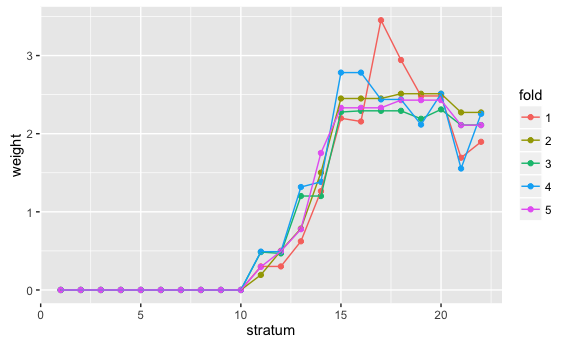We see that the general trend is driven by the covariate (stratum) and not as much by the fold. Recall that IHW assumes that the “optimal” weights should be a function of the covariate (and hence the stratum) only. Therefore, the weight functions calculated on random (overlapping) splits of the data should behave similarly, while there should be no trend driven by the folds. Also as expected, genes with very low baseMean count get assigned a weight of 0, while genes with high baseMean count get prioritized. ### 2.1.2 Diagnostic plot: raw versus adjusted p-values gg <- ggplot(as.data.frame(ihw_res), aes(x = pvalue, y = adj_pvalue, col = group)) + geom_point(size = 0.25) + scale_colour_hue(l = 70, c = 150, drop = FALSE) gg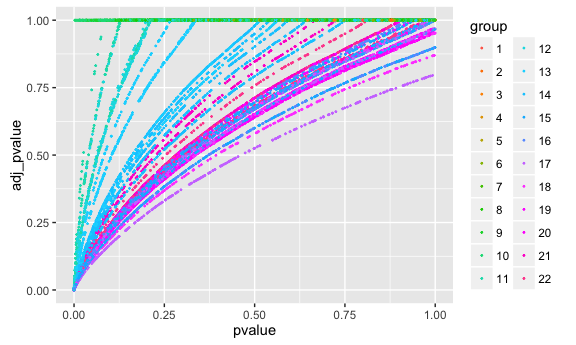gg %+% subset(as.data.frame(ihw_res), adj_pvalue <= 0.2)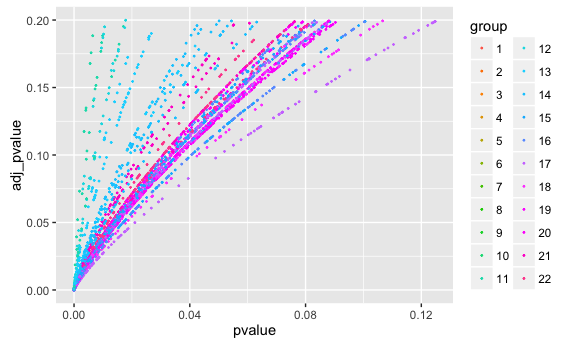As you can see above, the ihwResult object ihw_res can be converted to a data.frame, which contains the following columns: ihw_res_df <- as.data.frame(ihw_res) colnames(ihw_res_df) ##  "pvalue" "adj_pvalue" "weight" "weighted_pvalue" ##  "group" "covariate" "fold" ## 2.2 IHW for FWER control The standard IHW method presented above controls the FDR by using a weighted Benjamini-Hochberg procedure with data-driven weights. The same principle can be applied for FWER control by using a weighted Bonferroni procedure. Everything works exactly as above by using the keyword argument adjustment_type. For example: ihw_bonf <- ihw(pvalue ~ baseMean, data=de_res, alpha = 0.1, adjustment_type = "bonferroni") # 3 Choice of a covariate ## 3.1 Necessary criteria for choice of a covariate In which cases is IHW applicable? Whenever we have a covariate that is: 1. informative of power 2. independent of the p-values under the null hypothesis 3. not notably related to the dependence structure -if there is any- of the joint test statistics. ## 3.2 A few examples of such covariates Below we summarize some examples where such a covariate is available: • For row-wise $$t$$-tests we can use the overall (row-wise) variance (Bourgon, Gentleman, and Huber 2010). • For row-wise rank-based tests (e.g. Wilcoxon) we can use any function that does not depend on the order of arguments (Bourgon, Gentleman, and Huber 2010). • In DESeq2, we can use baseMean, as illustrated above (Love, Huber, and Anders 2014). • In eQTL analysis we can use the SNP-gene distance, the DNAse sensitivity, a HiC score, etc. (Ignatiadis et al. 2016). • In genome-wide association (GWAS), the allele frequency. • In quantitative proteomics with mass spectrometry, the number of peptides (Ignatiadis et al. 2016). ## 3.3 Why are the different covariate criteria necessary? The power gains of IHW are related to property 1, while its statistical validity relies on properties 2 and 3. For many practically useful combinations of covariates with test statistics, property 1 is easy to prove (e.g. through Basu’s theorem as in the $$t$$-test / variance example), while for others it follows by the use of deterministic covariates and well calibrated p-values (as in the SNP-gene distance example). Property 3 is more complicated from a theoretical perspective, but rarely presents a problem in practice – in particular, when the covariate is well thought out, and when the test statistics is such that it is suitable for the Benjamini Hochberg method without weighting. If one expects strong correlations among the tests, then one should take care to use a covariate that is not a driving force behind these correlations. For example, in genome-wide association studies, the genomic coordinate of each SNP tested is not a valid covariate, because the position is related to linkage disequilibrium (LD) and thus correlation among tests. On the other hand, in eQTL, the distance between SNPs and phenotype (i.e. transcribed gene) is not directly related to (i.e. does not increase or decrease) any potential correlations between test statistics, and thus is a valid covariate. ## 3.4 Diagnostic plots for the covariate Below we describe a few useful diagnostics to check whether the criteria for the covariates are applicable. If any of these are violated, then one should not use IHW with the given covariate. ### 3.4.1 Scatter plots To check whether the covariate is informative about power under the alternative (property 1), one should plot the p-values (or usually better, $$-log_{10}(\text{p-value})$$) against the ranks of the covariate: de_res <- na.omit(de_res) de_res$geneid <- as.numeric(gsub("ENSG[+]*", "", rownames(de_res)))

# set up data frame for plotting
df <- rbind(data.frame(pvalue = de_res$pvalue, covariate = rank(de_res$baseMean)/nrow(de_res),
covariate_type="base mean"),
data.frame(pvalue = de_res$pvalue, covariate = rank(de_res$geneid)/nrow(de_res),
covariate_type="gene id"))

ggplot(df, aes(x=covariate, y = -log10(pvalue))) +
geom_hex(bins = 100) +
facet_grid( . ~ covariate_type)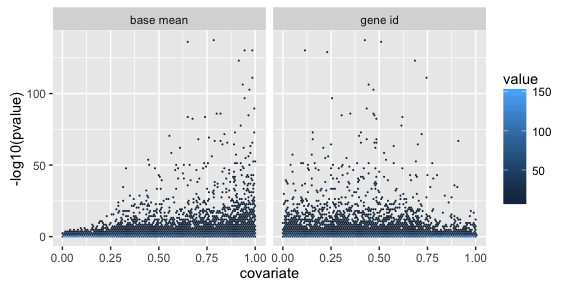On the left, we plotted $$-log_{10}(\text{p-value})$$ agains the (normalized) ranks of the base mean of normalized counts. This was the covariate we used in our DESeq2 example above. We see a clear trend: Low p-values are enriched at high covariate values. For very low covariate values, there are almost no small p-values. This indicates that the base mean covariate is correlated with power under the alternative.

On the other hand, the right plot uses a less useful statistic; the gene identifiers interpreted as numbers. Here, there is no obvious trend to be detected.

### 3.4.2 Stratified p-value histograms

One of the most useful diagnostic plots is the p-value histogram (before applying any multiple testing procedure). We first do this for our DESeq2 p-values:

ggplot(de_res, aes(x = pvalue)) + geom_histogram(binwidth = 0.025, boundary = 0)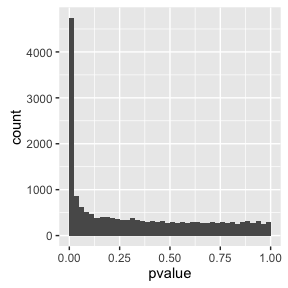This is a well calibrated histogram. As expected, for large p-values (e.g., for p-values $$\geq 0.5$$) the distribution looks uniform. This part of the histogram corresponds mainly to null p-values. On the other hand, there is a peak close to 0. This is due to the alternative hypotheses and can be observed whenever the tests have enough power to detect the alternative. In particular, in the airway dataset, as analyzed with DESeq2, we have a lot of power to detect differentially expressed genes. If you are not familiar with these concepts and more generally with interpreting p-value histograms, we recommend reading David Robinson’s blog post.

Now, when applying IHW with covariates, it is instrumental to not only check the histogram over all p-values, but also to check histograms stratified by the covariate.

Here we split the hypotheses by the base mean of normalized counts into a few strata and then visualize the conditional histograms:

de_res$baseMean_group <- groups_by_filter(de_res$baseMean, 8)

ggplot(de_res, aes(x=pvalue)) +
geom_histogram(binwidth = 0.025, boundary = 0) +
facet_wrap( ~ baseMean_group, nrow = 2)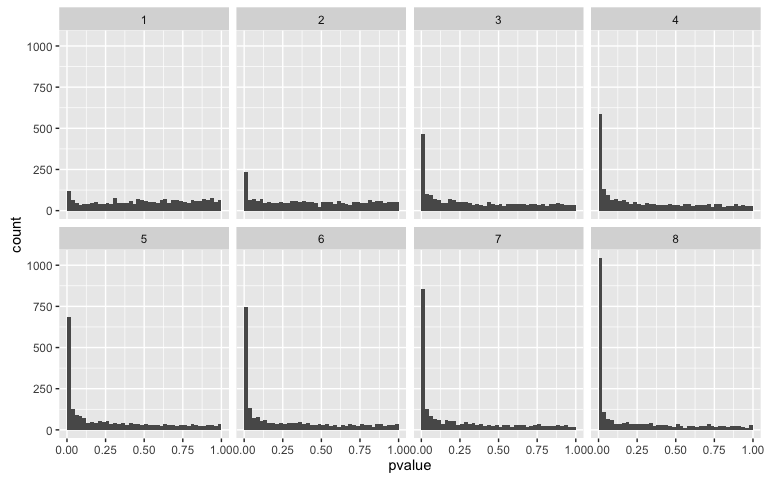Notice that all of these histograms are well calibrated, since all of them show a uniform distribution at large p-values. In many realistic examples, if this is the case, then IHW will control the FDR. Thus, this is a good check of whether properties 2 and 3 hold. In addition, these conditional histograms also illustrate whether property 1 holds: Notice that as we move to strata corresponding to higher mean counts, the peak close to 0 becomes taller and the height of the uniform tail becomes lower. This means that the covariate is associated with power under the alternative.

The empirical cumulative distribution functions (ECDF) offer a variation of this visualisation. Here, one should check whether the curves can be easily distinguished and whether they are almost linear for high p-values.

ggplot(de_res, aes(x = pvalue, col = baseMean_group)) + stat_ecdf(geom = "step")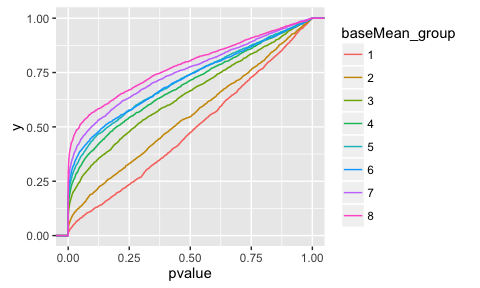Finally, as an example of an invalid covariate, we use the estimated log fold change. Of course, this is not independent of the p-values under the null hypothesis. We confirm this by plotting conditional histograms / ECDFs, which are not well calibrated:

de_res$lfc_group <- groups_by_filter(abs(de_res$log2FoldChange),8)

ggplot(de_res, aes(x = pvalue)) +
geom_histogram(binwidth = 0.025, boundary = 0) +
facet_wrap( ~ lfc_group, nrow=2)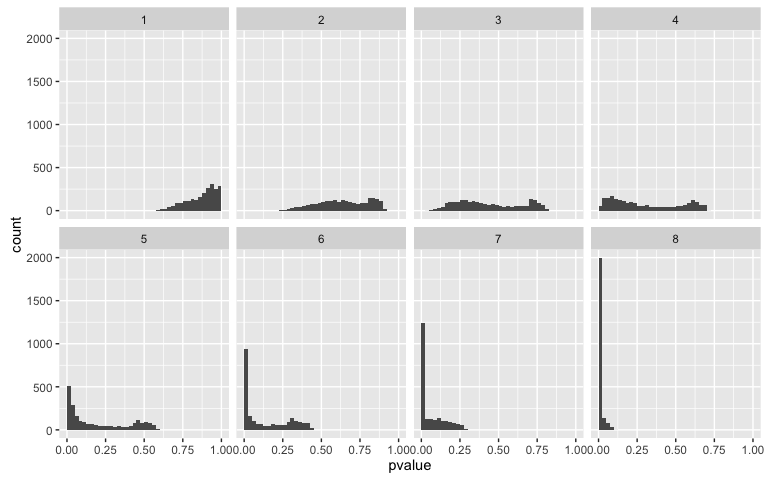ggplot(de_res, aes(x = pvalue, col = lfc_group)) + stat_ecdf(geom = "step")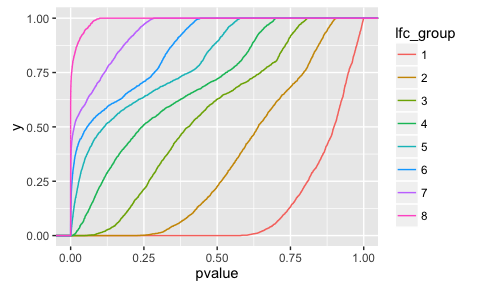For more details regarding choice and diagnostics of covariates, please also consult the Independent Filtering paper (Bourgon, Gentleman, and Huber 2010), as well as the genefilter vignettes.

# 4 Advanced usage: Working with incomplete p-value lists

So far, we have assumed that a complete list of p-values is available, i.e. one p-value per hypothesis. However, this information is not always available or practical:

• This can be related to the software tools used for the calculation of the p-values. For example, as noted in (Ochoa et al. 2015), some tools such as HMMER, only return the lowest p-values. In addition, other tools, such as MatrixEQTL (Shabalin 2012) by default only return p-values below a pre-specified threshold, for example all p-values below $$10^{-5}$$. In the case of HMMER, this is done because higher p-values are not reliable, while for MatrixEQTL it reduces storage requirements.
• Even if p-values for all hypotheses are available, explicit computation on them might exhaust the available computing resources (in particular, working memory).

Since rejections take place for low p-values (at the tails of the p-value distribution), we do not lose a lot of information by discarding the high p-values from the analysis, as long as we keep track of how many large p-values have been omitted. Thus, the above situations can be easily handled.

Before proceeding with the walkthrough for handling such cases with IHW, we quickly review how this is handled by p.adjust. We first simulate some data, where the power under the alternative depends on a covariate. p-values are calculated by a simple one-sided z-test.

set.seed(1)
X   <- runif(100000, min = 0, max = 2.5)      # covariate
H   <- rbinom(100000, size = 1, prob = 0.1)   # hypothesis true or false
Z   <- rnorm(100000, mean = H*X)              # Z-score
pvalue <- 1 - pnorm(Z)                        # pvalue
sim <- data.frame(X = X, H = H, Z = Z, pvalue = pvalue)

We can apply the Benjamini-Hochberg procedure to these p-values:

padj <- p.adjust(sim$pvalue, method="BH") sum( padj <= 0.1) ##  501 Now assume we only have access to the p-values $$\leq 0.1$$: filter_threshold <- 0.1 selected <- which(pvalue <= filter_threshold) pvalue_filt <- pvalue[selected] Then we can still use p.adjust, as long as we inform it of how many hypotheses were really tested (not just the ones with p-value $$\leq 0.1$$). We specify this by setting the n function argument. padj_filt <- p.adjust(pvalue_filt, method = "BH", n = length(pvalue)) qplot(padj[selected], padj_filt)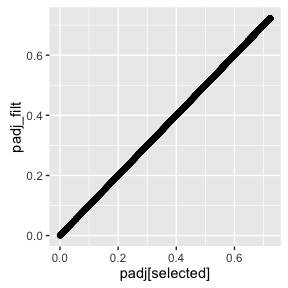sum(padj_filt <= 0.1)  ##  501 We see that we get exactly the same number of rejections, as when we used the whole p-value vector as input. Now, the same approach can be used with IHW, but is slighly more complicated. In particular, we need to provide information about how many hypotheses were conducted at each given value of the covariate. This means that there are two modifications to the standard IHW workflow: • If a numeric covariate is provided, IHW internally discretizes it and in this way bins the hypotheses into groups (strata). For the advanced functionality, this discretization has to be done manually by the user. In other words, the covariate provided by the user has to be a factor. For this, the convenience function groups_by_filter is provided, which returns a factor that stratifies a numeric covariate into a given number of groups with approximately the same number of hypotheses in each of the groups. This is a very simple function, largely equivalent to cut(., quantile(., probs=seq(0, 1, length.out=nbins)). • For the algorithm to work correctly, it is necessary to know the total number of hypotheses in each of the bins. However, if filtered p-values are used, IHW obviously cannot infer the number of hypotheses per bin automatically.Therefore, the user has to specify the number of hypotheses per bin manually via the m_groups option. (When there is only 1 bin, IHW reduces to BH and m_groups would be equivalent to the n keyword of p.adjust.) For example, when the whole grouping factor is available (e.g. when it was generated by using groups_by_filter on the full vector of covariates), then one can apply the table function on it to calculate the number of hypotheses per bin. This is then used as an input for the m_groups argument. More elaborate strategies might be needed in more complicated case, e.g. when the full vector of covariates can also not fit into RAM. nbins <- 20 sim$group <- groups_by_filter(sim$X, nbins) m_groups <- table(sim$group)

Now we can subset our data frame to only keep low p-values and then apply IHW with the manually specified m_groups.

sim_filtered <- subset(sim, sim\$pvalue <= filter_threshold)
ihw_filt <- ihw(pvalue ~ group, data=sim_filtered,
alpha = .1, m_groups = m_groups)
rejections(ihw_filt)
##  947